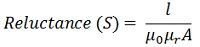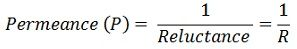# Magnetic Reluctance

Definition: The obstruction offered by a magnetic circuit to the magnetic flux is known as reluctance. As in electric circuit, there is resistance similarly in the magnetic circuit, there is a reluctance, but resistance in an electrical circuit dissipates the electric energy and the reluctance in magnetic circuit stores the magnetic energy.

Also in an electric circuit, the electric field provides the least resistance path to the electric current. Similarly, the magnetic field causes the least reluctance path for the magnetic flux. It is denoted by S.Where, l – the length of the conductor
μo – permeability of vacuum which is equal to 4π Χ107 Henry/metre.
μr – relative permeability of the material.
A – cross-section area of the conductor.

Its SI unit is AT / Wb (ampere-turns / Weber). The reluctance of the magnetic circuit is directly proportional to the length of the conductor and inversely proportional to the cross-section area of the conductor.

The reciprocal of the magnetic reluctance is known as the magnetic permeance. It is given by the expressionThe reluctance in the DC field is defined as the ratio of the magnetic motive force and to the magnetic flux of the same circuit. The reluctance in the DC field is expressed asWhere, S – reluctance in ampere-turns per weber.
F – magnetic motive force
Φ – magnetic flux

The non-uniform magnetic circuit is made by adding the uniform sections having the different value of a length, cross-section area, and permeability of the magnetic circuit.

The reluctance of the non-uniform circuit is calculated by adding the reluctance of the uniform section of the magnetic circuit. The calculation of the non-uniform magnetic field is more complex as compared to the uniform magnetic field.

In most of the transformer, an air gap is created for reducing the effects of the saturation. The air gap increases the reluctance of the circuit and hence stores more magnetic energy before the saturation.

### 2 thoughts on “Magnetic Reluctance”

1. Really your content is very good sir it had solved my doubt but I have one more doubt sir could you please explain it for me sir?and what my doubt is how can a magnetic circuit stores magnetic energy?I am eagerly waiting for your reply sir.